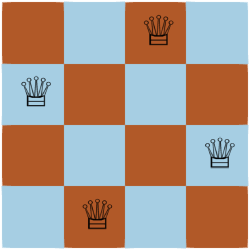# N-Queens

This tutorial was generated using Literate.jl. Download the source as a .jl file.

This tutorial was originally contributed by Matthew Helm and Mathieu Tanneau.

The N-Queens problem involves placing N queens on an N x N chessboard such that none of the queens attacks another. In chess, a queen can move vertically, horizontally, and diagonally so there cannot be more than one queen on any given row, column, or diagonal.Note that none of the queens above are able to attack any other as a result of their careful placement.

using JuMP
import HiGHS
import LinearAlgebra

N-Queens

N = 8

model = Model(HiGHS.Optimizer)
set_silent(model)

Next, let's create an N x N chessboard of binary values. 0 will represent an empty space on the board and 1 will represent a space occupied by one of our queens:

@variable(model, x[1:N, 1:N], Bin);

Now we can add our constraints:

There must be exactly one queen in a given row/column

for i in 1:N
@constraint(model, sum(x[i, :]) == 1)
@constraint(model, sum(x[:, i]) == 1)
end

There can only be one queen on any given diagonal

for i in -(N - 1):(N-1)
@constraint(model, sum(LinearAlgebra.diag(x, i)) <= 1)
@constraint(model, sum(LinearAlgebra.diag(reverse(x; dims = 1), i)) <= 1)
end

We are ready to put our model to work and see if it is able to find a feasible solution:

optimize!(model)

We can now review the solution that our model found:

solution = round.(Int, value.(x))
8×8 Matrix{Int64}:
0  0  0  0  1  0  0  0
0  0  1  0  0  0  0  0
0  0  0  0  0  0  0  1
0  0  0  1  0  0  0  0
0  0  0  0  0  0  1  0
1  0  0  0  0  0  0  0
0  0  0  0  0  1  0  0
0  1  0  0  0  0  0  0